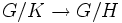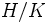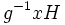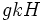# Difference between revisions of "Index is multiplicative"

## Statement

### Set-theoretic version

Suppose$K \le H \le G$ are groups. Then, we have a natural surjective map between the left coset spaces:$G/K \to G/H$

with the property that the inverse image of each point has size equals to the size of the left coset space$H/K$.

### Numerical version

Suppose$K \le H \le G$ are groups such that the indices$[G:H]$ and$[H:K]$ are finite. Then, we have:$[G:K] = [G:H][H:K]$.

Note that the statement makes sense even for infinite groups, if we interpret the cardinalities of the coset spaces as infinite cardinals and do the multiplication accordingly.

## Related facts

• Lagrange's theorem: Lagrange's theorem is a special case of this where$K$ is the trivial group.
• Third isomorphism theorem: The third isomorphism theorem is a stronger version of the statement where both subgroups are normal in the whole group. In this case, the surjective map from$G/K$ to$G/H$ is a homomorphism and the kernel is$H/K$.

## Facts used

1. Subgroup containment implies coset containment: If$K \le H \le G$ ,then every left coset of$K$ is contained in a unique left coset of$H$.

## Proof

### Proof of the set-theoretic version

Given: Groups$K \le H \le G$.

To prove: There is a surjective map from$G/K$ to$G/H$ where the inverse image of every point has size equal to the size of$H/K$.

Proof: Define$\varphi$ as the map sending a coset of$K$ to the unique coset of$H$ containing it (fact (1)). In other words:$\varphi(gK) = gH$.$\varphi$ is a well-defined map from$G/K$ to$G/H$. Further:

•$\varphi$ is surjective, since for any coset$gH$ of$H$ in$G$,$gH = \varphi(gK)$.
• The size of each inverse image equals the size of$H/K$: Consider a coset$gK$. We want to find all the left cosets of$H$ which map to this. This is equivalent to finding all the left cosets of$H$ contained in$gK$.

For this, consider a map$\psi$ that sends a left coset$xH$ of$H$ in$gK$, to the left coset$g^{-1}xH$.

Note that:

• This map$\psi$ is well-defined, because if$x$ is replaced by$xh, h \in H$, the left cosets$g^{-1}xH$ and$g^{-1}xhH$ are the same.
• The map$\psi$ sends left cosets of$H$ in$gK$ to left cosets of$H$ in$K$, because since$x \in gK$,$g^{-1}x \in K$.
• The map is injective, because it comes from a left multiplication on cosets.
• The map is surjective, because any left coset$kH$ of$H$ in$K$ arises as the image of the left coset$gkH$, which lies in$gK$.

Thus,$\psi$ is a bijection between the left cosets of$H$ in$gK$ and the left cosets of$H$ in$K$. Thus, the number of left cosets of$H$ in$gK$ equals the size of the left coset space$K/H$.

### Proof of the numerical version

The set-theoretic version shows that$G/K$ is the disjoint union of$|G/H|$ sets, each of size$|H/K|$. This yields:$|G/K| = |G/H||H/K|$.

By the definition of index of a subgroup, this yields:$[G:K] = [G:H][H:K]$.